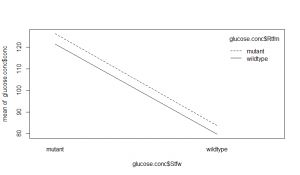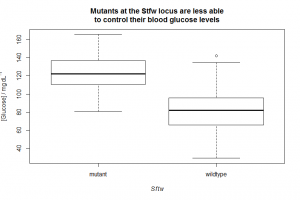# Analysis of variance: ANOVA (2 way)

The technique for a one-way ANOVA can be extended to situations where there is more than one factor, or – indeed – where there are several factors with several levels each, which may have synergistic or antagonistic effects on each other.

In the models we have seen so far (linear regression, one-way ANOVA) all we have really done is tested the difference between a null model (“y is a constant”, “y=“) and a single alternative model (“y varies by group” or “y=a+bx“) using an F test. However, in two-way ANOVA there are several possible models, and we will probably need to proceed through some model simplification from a complex model to a minimally adequate model.

The file wheat_yield.csv contains data on the yield (tn ha−1) of wheat from a large number of replicate plots that were either unfertilised, given nitrate alone, phosphate alone, or both forms of fertiliser. This requires a two-factor two-level ANOVA.

```wheat.yield<-read.csv( "H:/R/wheat_yield.csv" )
interaction.plot(
response     = wheat.yield\$yield,
x.factor     = wheat.yield\$N,
trace.factor = wheat.yield\$P
)```

As we’re plotting two factors, a box-and-whisker plot would make no sense, so instead we plot an interaction plot. It doesn’t particularly matter here whether we use `N`(itrate) as the `x.factor` (i.e. the thing we plot on the x-axis) and `P`(hosphate) as the `trace.factor` (i.e. the thing we plot two different trace lines for):You’ll note that the addition of nitrate seems to increase yield: both traces slope upwards from the `N`(o) to `Y`(es) level on the x-axis, which represents the nitrate factor. From the lower trace, it appears addition of just nitrate increases yield by about 1 tn ha−1.

You’ll also note that the addition of phosphate seems to increase yield: the `Y`(es) trace for phosphate is higher than the `N`(o) trace for phosphate. From comparing the upper and lower traces at the left (no nitrate), it appears that addition of just phosphate increases yield by about 2 tn ha−1.

Finally, you may notice there is a positive (synergistic) interaction. The traces are not parallel, and the top-right ‘point’ (`Y`(es) to both nitrate and phosphate) is higher than you would expect from additivity: the top-right is maybe 4, rather than 1+2=3 tn ha−1 higher than the completely unfertilised point.

We suspect there is an interaction, this interaction is biologically plausible, and we have 30 samples in each of the four treatments. We fit a two-factor (two-way) ANOVA maximal model, to see whether this interaction is significant.

First, we fit the model using `N*P` to fit the ‘product’ of the nitrate and phosphate factors, i.e.

```wheat.model<-aov(yield ~ N*P, data=wheat.yield )
anova( wheat.model )```
```Analysis of Variance Table
Response: yield
Df  Sum Sq Mean Sq  F value   Pr(>F)
N           1  83.645  83.645  43.3631   9.122e-10 ***
P           1 256.136 256.136 132.7859   < 2.2e-16 ***
N:P         1  11.143  11.143   5.7767   0.01759 *
Residuals 136 262.336   1.929
---
Signif. codes:
0 '***' 0.001 '**' 0.01 '*' 0.05 '.' 0.1 ' ' 1```

`N` shows the effect of nitrate, `P` the effect of phosphate, and `N:P` is the interaction term. As you can see, this interaction term appears to be significant: the nitrate+phosphate combination seems to give a higher yield than you would expect from just adding up the individual effects of nitrate and phosphate alone. To test this explicitly, we can fit a model that lacks the interaction term, using `N+P` to fit the ‘sum’ of the factors without the `N:P` term:

```wheat.model.no.interaction<-aov(yield ~ N+P, data=wheat.yield )
anova( wheat.model.no.interaction )```
```Analysis of Variance Table
Response: yield
Df  Sum Sq Mean Sq F value    Pr(>F)
N          1  83.645  83.645  41.902    1.583e-09 ***
P          1 256.136 256.136 128.312    < 2.2e-16 ***
Residuals 137 273.479   1.996
---
Signif. codes:
0 '***' 0.001 '**' 0.01 '*' 0.05 '.' 0.1 ' ' 1```

We can then use the `anova()` function with two arguments to compare these two models with an F test:

`anova( wheat.model, wheat.model.no.interaction )`
```Analysis of Variance Table
Model 1: yield ~ N * P
Model 2: yield ~ N + P
Res.Df    RSS Df Sum of Sq      F  Pr(>F)
1    136 262.34
2    137 273.48 -1   -11.143 5.7767 0.01759 *
---
Signif. codes:
0 '***' 0.001 '**' 0.01 '*' 0.05 '.' 0.1 ' ' 1```

An alternative way of doing the same thing is to update the maximal model by deletion. First you fit the maximal model, as before:

```wheat.model<-aov(yield ~ N*P, data=wheat.yield )
anova( wheat.model )```

You note the least significant term (largest p value) is the `N:P` term, and you selectively remove that from the model to produce a revised model with `update()`:

```wheat.model.no.interaction<-update( wheat.model, ~.-N:P)
anova( wheat.model, wheat.model.no.interaction )```

The `~.-N:P` is shorthand for “fit (~) the current `wheat.model` (.) minus () the interaction term (N:P)”. This technique is particularly convenient when you are iteratively simplifying a model with a larger number of factors and a large number of interactions.

Whichever method we use, we note that the deletion of the interaction term significantly reduces the explanatory power of the model, and therefore that our minimally adequate model is the one including the interaction term.

If you have larger numbers of factors (say `a`, `b` and `c`) each with a large number of levels (say 4, 2, and 5), it is possible to fit a maximal model (`y~a*b*c`), and to simplify down from that. However, fitting a maximal model in this case would involve estimating 40 separate parameters, one for each combination of the 4 levels of `a`, with the 2 levels of `b`, and the 5 levels of `c`. It is unlikely that your data set is large enough to make a model of 40 parameters a useful simplification compared to the raw data set itself. It would even saturate it if you have just one datum for each possible {a,b,c} combination. Remember that one important point of a model is to provide a simplification of a large data set. If you want to detect an interaction between factors `a` and `b` reliably, you need enough data to do so. In an experimental situation, this might impact on whether you actually want to make `c` a variable at all, or rather to control it instead, if this is possible.

# Exercises

Analyse the following data set with ANOVA

1. The file glucose_conc.csv contains fasting blood serum glucose levels (mg dL−1) for humans homozygous for either a mutant allele or the wild-type allele at two loci (Rtfm and Stfw) thought to be involved in blood glucose control. Do the data support a correlation between possession of the mutant alleles and elevated glucose levels?

1. Glucose concentrations
```glucose.conc<-read.csv( "H:/R/glucose_conc.csv" )
interaction.plot(
response     = glucose.conc\$conc,
x.factor     = glucose.conc\$Stfw,
trace.factor = glucose.conc\$Rtfm
)```The lines are parallel, so there seems little evidence of interaction between the loci. The difference between the mutant and wildtypes for the Rtfm locus doesn’t look large, and may not be significant. However, the Stfw wildtypes seem to have better control of blood glucose. A two-way ANOVA can investigate this:

```glucose.model<-aov( conc ~ Stfw*Rtfm, data = glucose.conc )
anova( glucose.model )```
```Analysis of Variance Table
Response: conc
Df Sum Sq Mean Sq  F value  Pr(>F)
Stfw        1 133233  133233 311.4965 < 2e-16 ***
Rtfm        1   1464    1464   3.4238 0.06526 .
Stfw:Rtfm   1     17      17   0.0407 0.84020
Residuals 296 126605     428
---
Signif. codes:
0 '***' 0.001 '**' 0.01 '*' 0.05 '.' 0.1 ' ' 1```

As we suspected, the `Stfw:Rtfm` interaction is not significant. We will remove it by deletion:

```glucose.model.no.interaction<-update( glucose.model, ~.-Stfw:Rtfm )
anova( glucose.model, glucose.model.no.interaction )```
```Analysis of Variance Table

Model 1: conc ~ Stfw * Rtfm
Model 2: conc ~ Stfw + Rtfm
Res.Df    RSS Df Sum of Sq      F Pr(>F)
1    296 126605
2    297 126622 -1   -17.421 0.0407 0.8402```

The p value is much larger than 0.05, so the model including the interaction term is not significantly better than the one excluding it.

The reduced model is now:

`anova(glucose.model.no.interaction )`
```Analysis of Variance Table
Response: conc
Df Sum Sq Mean Sq  F value  Pr(>F)
Stfw        1 133233  133233 312.5059 < 2e-16 ***
Rtfm        1   1464    1464   3.4349 0.06482 .
Residuals 297 126622     426
---
Signif. codes:
0 '***' 0.001 '**' 0.01 '*' 0.05 '.' 0.1 ' ' 1```

When we remove the interaction term, its variance is redistributed to the remaining factors, which will change their values compared to the maximal model we fitted at the start. It appears that the wildtype and mutants of Rtfm do not significantly differ in their glucose levels, so we will now remove that term too:

```glucose.model.stfw.only<-update( glucose.model.no.interaction, ~.-Rtfm )
anova( glucose.model.no.interaction, glucose.model.stfw.only )```
```Analysis of Variance Table
Model 1: conc ~ Stfw + Rtfm
Model 2: conc ~ Stfw
Res.Df    RSS Df Sum of Sq      F  Pr(>F)
1    297 126622
2    298 128087 -1   -1464.4 3.4349 0.06482 .
---
Signif. codes:
0 '***' 0.001 '**' 0.01 '*' 0.05 '.' 0.1 ' ' 1```

Again, we detect no significant different between the models, so we accept the simpler one (Occam’s razor).

`anova( glucose.model.stfw.only )`
```Analysis of Variance Table

Response: conc
Df Sum Sq Mean Sq F value    Pr(>F)
Stfw        1 133233  133233  309.97 < 2.2e-16 ***
Residuals 298 128087     430
---
Signif. codes:
0 '***' 0.001 '**' 0.01 '*' 0.05 '.' 0.1 ' ' 1```

The Stfw locus does have a very significant effect on blood glucose levels. We could try deletion testing Stfw too, but here it in not really necessary as the ANOVA table above is comparing the Stfw only model to the null model in any case. To determine what the effect of Stfw is, we can use a Tukey test:

`TukeyHSD( glucose.model.stfw.only )`
```  Tukey multiple comparisons of means
95% family-wise confidence level
Fit: aov(formula = conc ~ Stfw, data = glucose.conc)
\$Stfw
wildtype-mutant -42.14783 -46.859 -37.43666     0```

… or, as this model has in fact simplified down to the two-levels of one factor, this is equivalent to just doing a t test at this point:

`t.test( conc ~ Stfw, data = glucose.conc)`
```        Welch Two Sample t-test
data:  conc by Stfw
t = 17.6061, df = 289.288, p-value < 2.2e-16
alternative hypothesis: true difference in means is not equal to 0
95 percent confidence interval:
37.43609 46.85958
sample estimates:
mean in group mutant mean in group wildtype
123.87824               81.73041```

Given this analysis, the best way to represent our data is probably a simple boxplot ignoring Rtfm, with an explanatory legend:

```boxplot(
conc ~ Stfw,
data = glucose.conc,
xlab = expression( italic(Sftw) ),
ylab = expression( "[Glucose] / mg "*dL^-1 ),
main = "Mutants at the Stfw locus are less able\nto control their blood glucose levels"
)```Homozygous mutants at the Stfw locus were found to be less well able to control their blood glucose levels (F=310, p≪0.001). Homozygous mutation of the Rtfm locus was found to have no significant effect on blood glucose levels (F on deletion from ANOVA model = 3.4, p=0.06). Homozygous mutation at the Stfw locus was associated with a blood glucose level 40 mg dL−1 (95% CI: 37.4…46.8) higher than the wildtype (t=17.6, p≪0.001).

Next up… Nonlinear regression.

This site uses Akismet to reduce spam. Learn how your comment data is processed.